SLVSCE1C August   2014  – November 2015

PRODUCTION DATA.

1. Features
2. Applications
3. Description
4. Application Schematic
5. Revision History
6. Pin Configuration and Functions
7. Specifications
8. Parametric Measurement Information
9. Detailed Description
1. 9.1 Overview
2. 9.2 Functional Block Diagram
3. 9.3 Feature Description
4. 9.4 Device Functional Modes
10. 10Applications and Implementation
1. 10.1 Application Information
2. 10.2 Typical Application
1. 10.2.1 Precision Current Limiting and Protection for White Goods
3. 10.3 System Examples
11. 11Power Supply Recommendations
12. 12Layout
13. 13Device and Documentation Support
14. 14Mechanical, Packaging, and Orderable Information

• D|8

## 7 Specifications

### 7.1 Absolute Maximum Ratings

over operating free-air temperature range (unless otherwise noted) (1)
VALUE(2) UNIT
MIN MAX
Input voltage range IN, OUT, ENUV, OVP, FLT –0.3 20 V
IN (10 ms Transient) 22
ILIM, SS –0.3 7
Sink current SS 5 mA
FLT 100 mA
Source current ILIM, SS, FLT Internally Limited
Maximum junction temperature, TJ Internally Limited °C
Storage temperature range, Tstg -65 150 °C
(1) Stresses beyond those listed under absolute maximum ratings may cause permanent damage to the device. These are stress ratings only and functional operation of the device at these or any conditions beyond those indicated under recommended operating conditions is not implied. Exposure to absolute-maximum-rated conditions for extended periods may affect device reliability.
(2) All voltage values, except differential voltages, are with respect to network ground terminal.

### 7.2 ESD Ratings

VALUE UNIT
V(ESD) Electrostatic discharge Human body model (HBM), per ANSI/ESDA/JEDEC JS-001(1) ±2000 V
Charged device model (CDM), per JEDEC specification JESD22-C101(2) ±500
(1) JEDEC document JEP155 states that 500-V HBM allows safe manufacturing with a standard ESD control process.
(2) JEDEC document JEP157 states that 250-V CDM allows safe manufacturing with a standard ESD control process.

### 7.3 Recommended Operating Conditions

over operating free-air temperature range (unless otherwise noted)
MIN TYP MAX UNIT
Input voltage range IN 4.5 18 V
OUT, OVP, ENUV, FLT 0 18
SS 0 6
ILIM 0 3.3
Resistance ILIM 35.7 95.3 158
External capacitance OUT 0.1 1 µF
SS 1 1000 nF
Operating junction temperature range, TJ –40 25 125 °C

### 7.4 Thermal Characteristics(1)

THERMAL METRIC TPS2592xx UNIT
SOIC (8) PINS
RθJA Junction-to-ambient thermal resistance 120.8 °C/W
RθJCtop Junction-to-case (top) thermal resistance 65.5
RθJB Junction-to-board thermal resistance 51.8
ψJT Junction-to-top characterization parameter 17.4
ψJB Junction-to-board characterization parameter 61.2

### 7.5 Electrical Characteristics

Conditions (unless otherwise noted) are –40°C ≤ TJ ≤ 125°C, 4.5 V ≤ V(IN) ≤ 18 V, V(EN UV) = 2 V, V(OVP) = 0 V, R(ILIM) = 95.3 kΩ, CSS = OPEN, FLT = OPEN. Positive current into terminals. All voltages are referenced to GND (unless otherwise noted).
PARAMETER TEST CONDITIONS MIN TYP MAX UNIT
SUPPLY VOLTAGE AND INTERNAL UNDERVOLTAGE LOCKOUT
V(IN) Operating Input Voltage 4.5 18 V
V(UVR) UVLO Threshold, Rising 4.10 4.26 4.40 V
V(UVHys) UVLO Hysteresis 168 224 279 mV
IQ( ON) Supply Current, Enabled V(ENUV) = 2 V, V(IN) = 12 V 0.22 0.41 0.58 mA
IQ( OFF) Supply Current, Disabled V(ENUV) = 0 V, V(IN) = 12 V 0.08 0.132 0.20 mA
OVERVOLTAGE PROTECTION (OVP) INPUT
V(OVPR) Overvoltage Threshold Voltage, Rising 1.35 1.39 1.43 V
V(OVPF) Overvoltage Threshold Voltage, Falling 1.30 1.34 1.37 V
I(OVP) OVP Input Leakage Current 0V ≤ V(OVP) ≤ 18 V –100 0 100 nA
ENABLE AND UNDERVOLTAGE LOCKOUT (ENUV) INPUT
V(ENR) ENUV Threshold voltage, rising 1.36 1.39 1.42 V
V(ENF) ENUV Threshold voltage, falling 1.30 1.34 1.37 V
V(ENF_RST) ENUV Threshold voltage to reset thermal fault, falling 0.5 0.61 0.8 V
IEN EN Input leakage current 0 ≤ V(ENUV) ≤ 18 V –100 0 100 nA
SOFT START: OUTPUT RAMP CONTROL (SS)
I(SS) SS charging current V(SS) = 0 V 0.9 1.04 1.2 µA
R(SS) SS discharging resistance V(ENUV) = 0 V, I(SS) = 10 mA sinking 60 70 85 Ω
V(SSmax) SS maximum capacitor voltage 5.5 V
GAIN(SS) SS to OUT gain ΔV(OUT)/ΔV(SS) 4.81 4.86 4.92 V/V
CURRENT LIMIT PROGRAMMING (ILIM)
I(ILIM) ILIM Bias current 6 10 16 µA
ILIMIT Current Limit(2) R(ILIM) = 35.7 kΩ, (V(IN) - V(OUT)) = 1 V 0.284 0.368 0.452 A
R(ILIM) = 45.3 kΩ, (V(IN) - V(OUT)) = 1 V 0.394 0.471 0.547
R(ILIM) = 95.3 kΩ, (V(IN) - V(OUT)) = 1 V, TA = TJ= 25°C 0.98 1.0 1.02
R(ILIM) = 95.3 kΩ, (V(IN) - V(OUT)) = 1 V 0.93 1.0 1.062
R(ILIM) = 150 kΩ, (V(IN) - V(OUT)) = 1 V 1.43 1.57 1.7
R(ILIM) = SHORT, Shorted resistor current limit
R(ILIM) = OPEN, Open resistor current limit
(Single Point Failure Test: UL60950)
0.12 0.257 0.406
IOS Short-circuit current limit(2) R(ILIM) = 35.7 kΩ, (V(IN) - V(OUT)) = 12 V 0.275 0.356 0.438 A
R(ILIM) = 45.3 kΩ, (V(IN) - V(OUT)) = 12 V 0.376 0.45 0.522
R(ILIM) = 95.3 kΩ, (V(IN) - V(OUT)) = 12 V 0.837 0.9 0.964
R(ILIM) = 150 kΩ, (V(IN) - V(OUT)) = 12 V 1.219 1.34 1.46
I(FASTRIP) Fast-Trip comparator threshold R(ILIM) in kΩ 0.0142 x R(ILIM) + 0.36 A
V(ILIMopen) ILIM Open resistor detect threshold V(ILIM) Rising, R(ILIM) = OPEN 2.81 3.0 3.25 V
MOSFET – POWER SWITCH
RDS(on)

FET ON resistance(1)

–40°C ≤ TJ ≤ 85°C 55 87 120
–40°C ≤ TJ ≤ 125°C 55 87 135
PASS FET OUTPUT (OUT)
Ilkg(OUT) OUT Bias current in off state V(ENUV) = 0 V, V(OUT) = 0 V (Sourcing) –2 0 1 µA
Isink(OUT) V(ENUV) = 0V, V(OUT) = 300 mV (Sinking) 5 7 10
FAULT FLAG (FLT): ACTIVE LOW
R(FLT) FLT Pull down Resistance Device in fault condition, V(ENUV) = 0V, I(FLT) = 100mA 22 26 32 Ω
I(FLT) FLT Input Leakage Current Device not in fault condition, V(FLT) = 0V, 18V –0.5 0 0.5 µA
THERMAL SHUT DOWN (TSD)
T(TSD) TSD Threshold, rising(1) 155 °C
T(TSDhys) TSD Hysteresis(1) 20 °C
Thermal fault: Latched or Auto Retry TPS25921L LATCHED
TPS25921A AUTO-RETRY
(1) The limits for these parameters are specified based on design and characterization data, and are not tested during production.
(2) Pulse-testing techniques maintain junction temperature close to ambient temperature. Thermal effects must be taken into account separately.

### 7.6 Timing Requirements

Conditions (unless otherwise noted) are –40°C ≤ TJ ≤ 125°C, V(IN)= 12 V, V(EN UV) = 2 V, V(OVP) = 0 V, R(ILIM) = 95.3 kΩ, CSS = OPEN, FLT = OPEN. Positive current into terminals. All voltages are referenced to GND (unless otherwise noted). Refer to Figure 26 for the timing diagrams
MIN TYP MAX UNIT
ENABLE AND UNDERVOLTAGE LOCKOUT (ENUV) INPUT
tOFF(dly) Turn Off delay ENUV ↓ to V(OUT) 8 µs
tON(dly) Turn-On delay ENUV ↑ to V(OUT) = 1V,
C(SS) = OPEN
96 µs
ENUV ↑ to V(OUT) = 1V,
C(SS) > 0.39nF, [C(dVdT) in nF]
14.5 + 0.5 x
(70p + C(SS))
OVERVOLTAGE PROTECTION (OVP) INPUT
tOVP(dly) OVP Disable delay OVP↑ to V(OUT) 8 µs
SOFT START: OUTPUT RAMP CONTROL (SS)
tSS Output ramp time ENUV ↑ to V(OUT) = 11 V, with C(SS) = open,
C(OUT) = 2.2 µF
0.2 0.26 0.33 ms
ENUV ↑ to V(OUT) = 11 V, with C(SS) = 1.2 nF,
C(OUT) = 2.2 µF
2.1 3 3.6
CURRENT LIMIT PROGRAMMING (ILIM)
tFASTRIP(dly) Fast-Trip comparator delay I(OUT) > I(FASTRIP) 3 µs
THERMAL SHUT DOWN (TSD)
tTSD(dly) Retry Delay after TSD recovery, TJ < [T(TSD) - 20oC] TPS25921A Only, V(IN) = 12 V 150 ms
TPS25921A Only, V(IN) = 4.5 V 100 ms

### 7.7 Typical Characteristics

Conditions (unless otherwise noted) are –40°C ≤ TJ ≤ 125°C, V(IN)= 12 V, V(EN UV) = 2 V, V(OVP) = 0 V, R(ILIM) = 95.3 kΩ, C(OUT) = 2.2 μF, CSS = OPEN, FLT = OPEN. Positive current into terminals. All voltages referenced to GND (unless otherwise noted). For all oscilloscope waveforms TA = 25°C.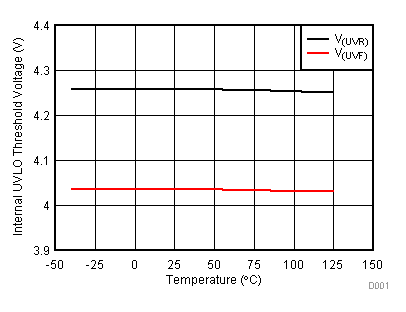Figure 1. UVLO Threshold Voltage vs Temperature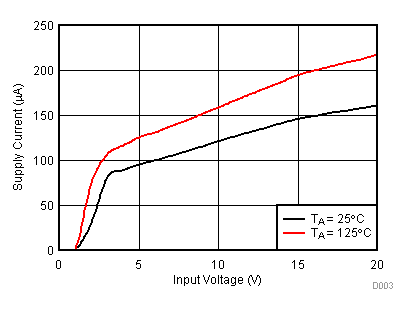Figure 3. Input Supply Current vs Supply Voltage at Shutdown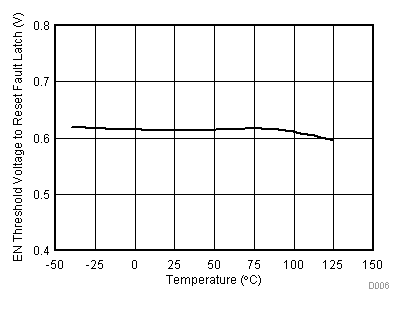Figure 5. EN Threshold Voltage to Reset Fault Latch vs Temperature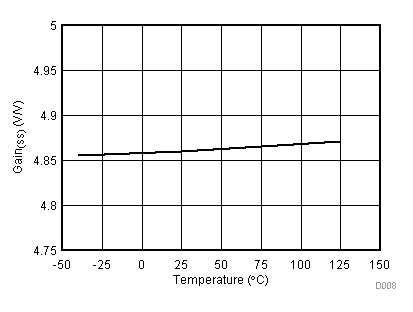Figure 7. GAIN(SS) vs Temperature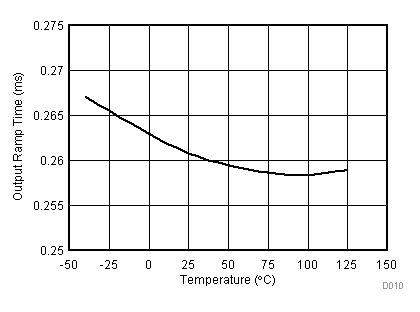C(SS) = Open
Figure 9. Output Ramp Time vs Temperature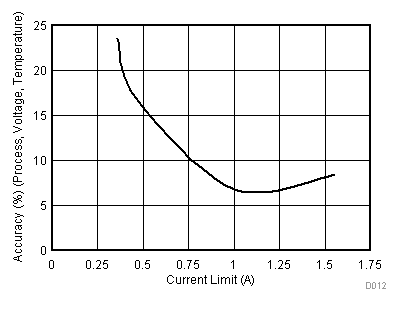Figure 11. Current Limit Accuracy vs Current Limit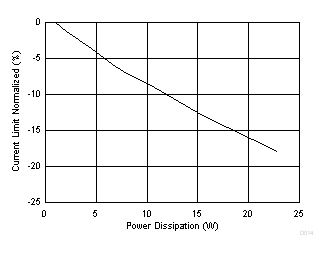PD = [V(IN)-V(OUT)]*ILIMIT
Figure 13. Current Limit Normalized (%) vs Power Dissipation in the Device PD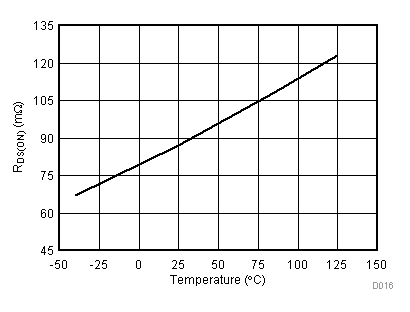Figure 15. RDS(ON) vs Temperature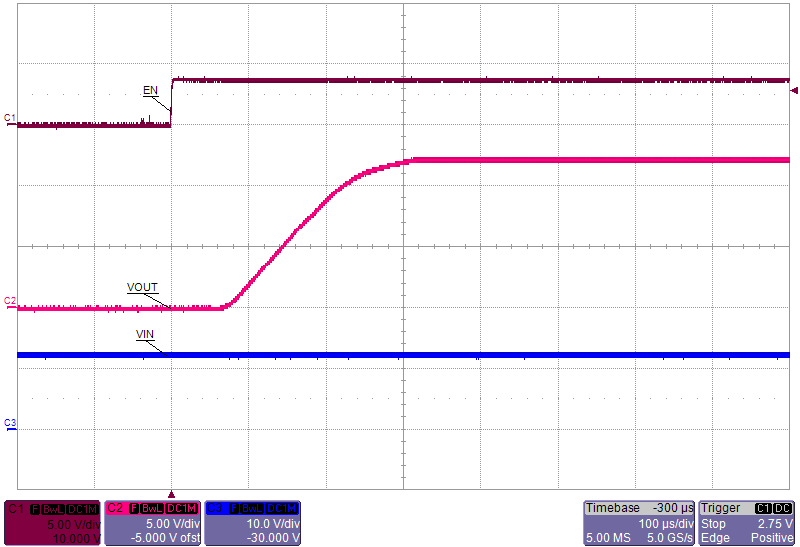C(SS) = Open C(OUT) = 4.7 nF
Figure 17. Turn ON with Enable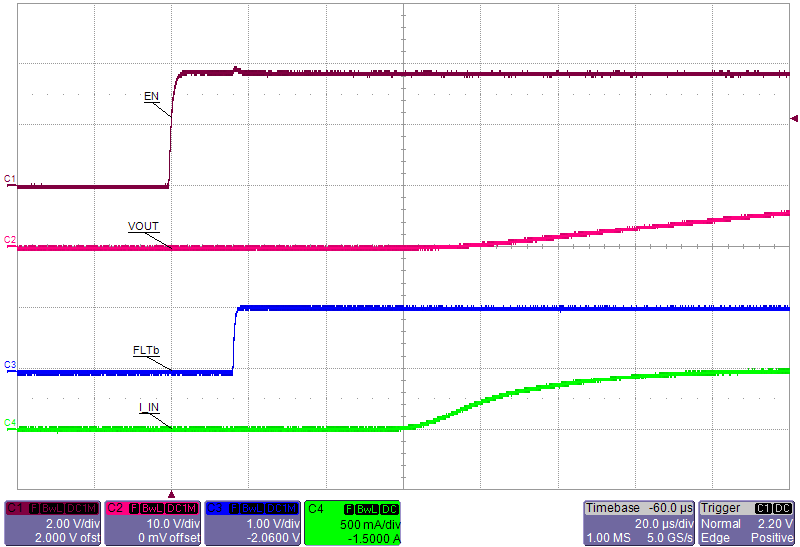R(FLT) = 100 kΩ
Figure 19. EN Turn ON Delay : EN ↑ to Output Ramp ↑RL = 12 Ω R(FLT) = 100 kΩ
Figure 21. OVP Turn OFF delay: OVP ↑ to Fault ↓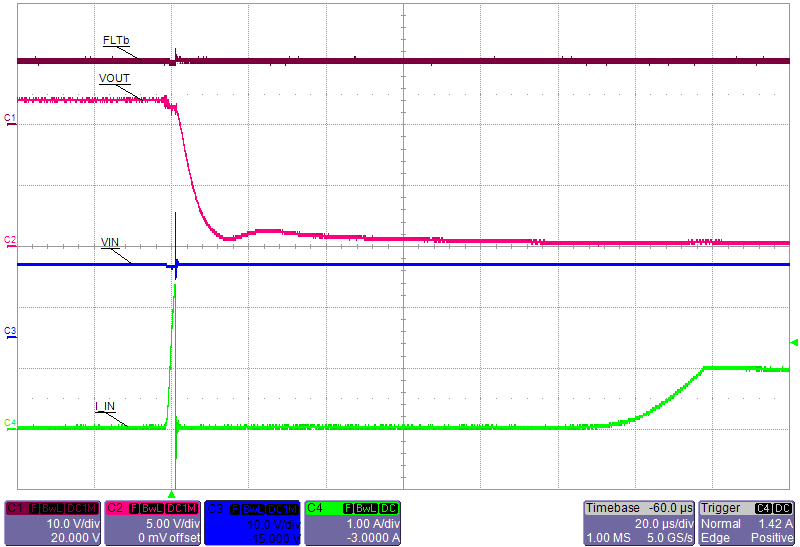Figure 23. Hot-Short: Fast Trip Response and Current Regulation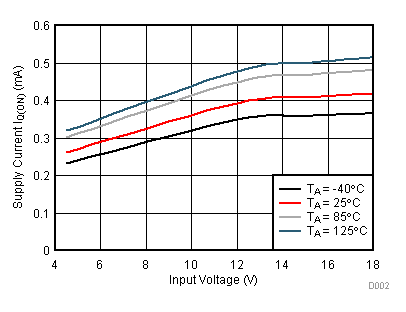Figure 2. Input Supply Current vs Supply Voltage During Normal OperationFigure 4. ENUV and OVP Threshold Voltage vs Temperature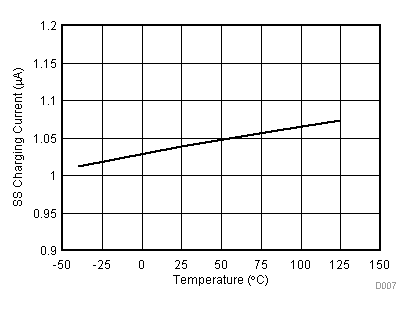Figure 6. SS Pin Charging Current vs Temperature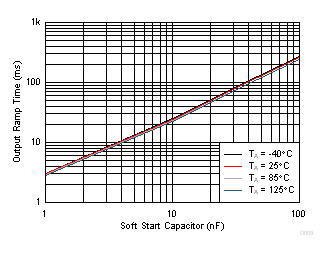Figure 8. Output Ramp Time vs C(SS)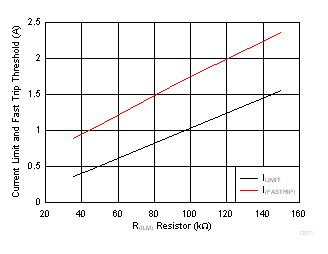Figure 10. Current Limit vs Current Limit Resistor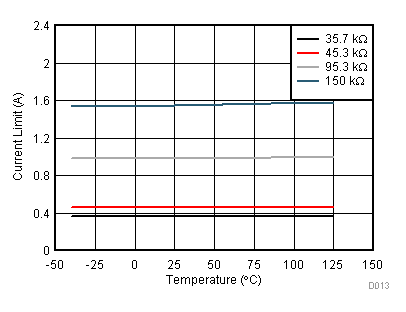Figure 12. Current Limit vs Temperature Across R(ILIM)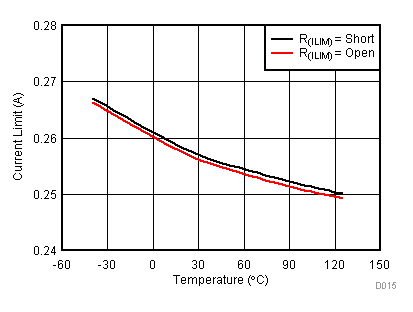Figure 14. Current Limit for R(ILIM) = Open and Short vs Temperature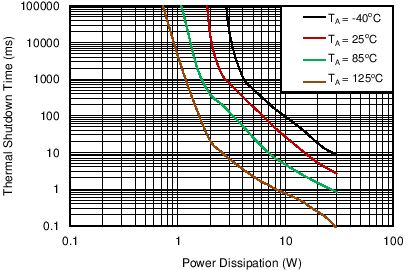Taken on 1-Layer board, 2oz.(0.08-mm thick) with GND plane area: 14 cm2 (bottom)
Figure 16. Thermal Shutdown Time vs Power Dissipation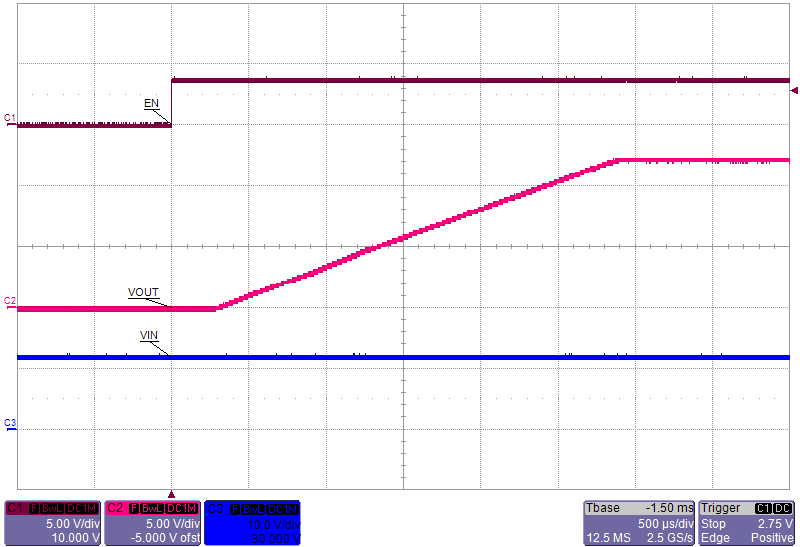C(SS) = 1nF C(OUT) = 4.7 nF
Figure 18. Turn ON with Enable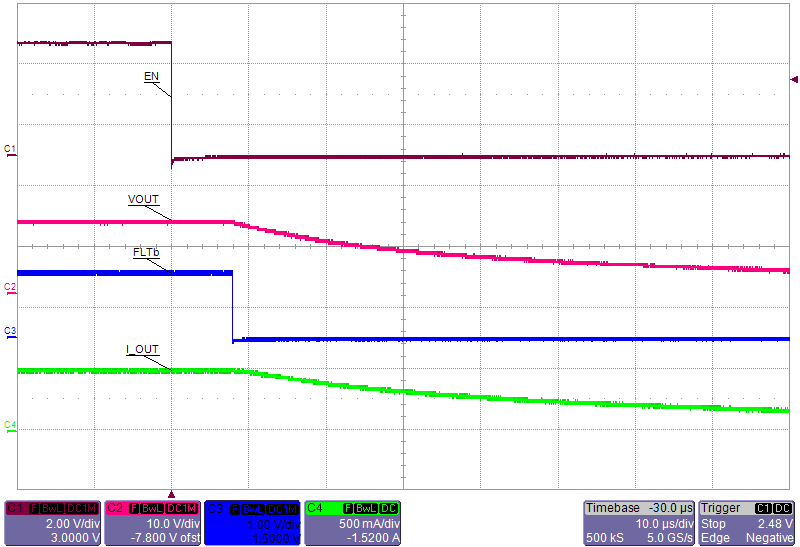R(FLT) = 100 kΩ
Figure 20. EN Turn OFF Delay : EN ↓ to Fault ↓RL = 12 Ω R(FLT) = 100 kΩ
Figure 22. OVP Turn ON delay: OVP ↓ to Output Ramp ↑Figure 24. Hot-Short: Fast Trip Response (Zoomed)